Practice the questions of McGraw Hill Math Grade 4 Answer Key PDF Chapter 9 Test to secure good marks & knowledge in the exams.

Multiply by 10 to find an equivalent fraction for each.

Question 1.
$$\frac{5}{10}$$
$$\frac{5}{10}$$ × $$\frac{10}{10}$$ = $$\frac{50}{10}$$

Question 2.
$$\frac{3}{10}$$
$$\frac{3}{10}$$ × $$\frac{10}{10}$$ = $$\frac{30}{10}$$

Question 3.
$$\frac{8}{10}$$
$$\frac{8}{10}$$ × $$\frac{10}{10}$$ = $$\frac{80}{10}$$

Divide by 10 to find an equivalent fraction for each.

Question 4.
$$\frac{20}{100}$$
$$\frac{20}{100}$$ ÷ $$\frac{10}{10}$$ = $$\frac{2}{10}$$

Question 5.
$$\frac{10}{100}$$
$$\frac{10}{100}$$ ÷ $$\frac{10}{10}$$ = $$\frac{1}{10}$$

Question 6.
$$\frac{70}{100}$$
$$\frac{70}{100}$$ ÷ $$\frac{10}{10}$$ = $$\frac{7}{10}$$

Question 7.
$$\frac{1}{10}$$ + $$\frac{3}{10}$$ = ____________
The denominators of the given fractions are same so add the numerators.
$$\frac{1}{10}$$ + $$\frac{3}{10}$$ = $$\frac{4}{10}$$ = $$\frac{2}{5}$$

Question 8.
$$\frac{46}{100}$$ + $$\frac{25}{100}$$ = ____________
The denominators of the given fractions are same so add the numerators.
$$\frac{46}{100}$$ + $$\frac{25}{100}$$ = $$\frac{71}{100}$$

Question 9.
$$\frac{4}{10}$$ + $$\frac{17}{100}$$ = ____________
$$\frac{4}{10}$$ × $$\frac{10}{10}$$ = $$\frac{40}{10}$$
$$\frac{40}{10}$$ + $$\frac{17}{100}$$ = $$\frac{57}{100}$$

Plot each decimal point or fraction on the number line provided.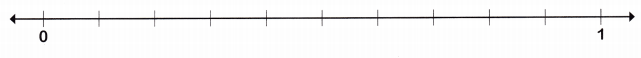Question 10.
Plot $$\frac{3}{5}$$ on the number line. Label it A.
$$\frac{3}{5}$$ = 0.6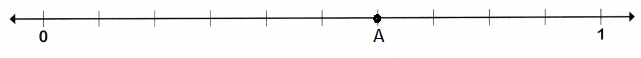Question 11.
Plot 0.20 on the number line. Label it B.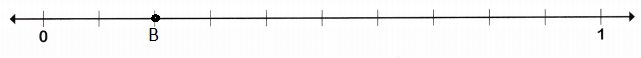Question 12.
Plot $$\frac{5}{10}$$ on the number line. Label it C.
$$\frac{5}{10}$$ = $$\frac{1}{2}$$ = 0.5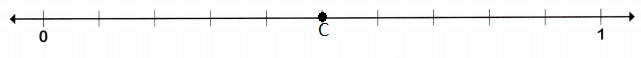Solve.

Question 13.
Look at the drawing below. Pedro begins at the starting point and hikes 1.2 miles. Where on the drawing does he stop?0.4 × 3 = 1.2 miles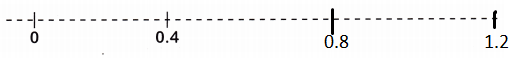Write each fraction or mixed number as a decimal.

Question 14.
$$\frac{6}{10}$$
$$\frac{6}{10}$$ in the decimal form can be written as 0.6

Question 15.
2$$\frac{78}{100}$$
2$$\frac{78}{100}$$ = $$\frac{278}{100}$$
$$\frac{278}{100}$$ in the decimal form can be written as 2.78

Question 16.
42$$\frac{7}{10}$$
42$$\frac{7}{10}$$ = $$\frac{427}{10}$$ = 42.7
42$$\frac{7}{10}$$ in the decimal form can be written as 42.7

Write the value of each underlined digit.

Question 17.
1.25
The place value of underlined digit 5 is 5 hundredths

Question 18.
0.48
The place value of underlined digit 4 is 4 tenths.

Question 19.
3.56
The place value of underlined digit 3 is the whole number.

Compare. Write <, >, or = for each.

Question 20.
0.41 ___________ 0.14
0.41 is greater than 0.14
0.41 > 0.14

Question 21.
1.72 ____________ 1.72
1.72 is equal to 1.72
1.72 = 1.72

Question 22.
3.81 ____________ 3.85
3.81 is less than 3.85
3.81 < 3.85

Write the amount with a dollar sign and decimal point.

Question 23.
1 dollar + 4 dimes + 7 pennies
1 dime = $0.10 1 penny =$0.01
$1 +$0.10 + $0.01 =$1.11

Question 24.
2 dollars + 5 pennies
1 penny = $0.01$2 + $0.05 =$2.05

Solve.

Question 25.
Benjamin and Bob went to the beach. Benjamin drew a line in the sand. It measured 3.48 feet. Bob drew a line that measured 2.39 yards. Benjamin says that since 3.48 is greater than 2.39, his line is longer. Do you agree? Why or why not?
Given,
Benjamin and Bob went to the beach. Benjamin drew a line in the sand.
It measured 3.48 feet.
3.48 ft = 1.16 yard
Bob drew a line that measured 2.39 yards.
1.16 yards is less than 2.39 yards
Benjamin says that since 3.48 is greater than 2.39
No, Benjamin is incorrect.

Question 26.
A German shepherd named Mindy weighs 74.09 pounds. Another German shepherd weighs 74.32 pounds. Would comparing these weights be valid? Why?